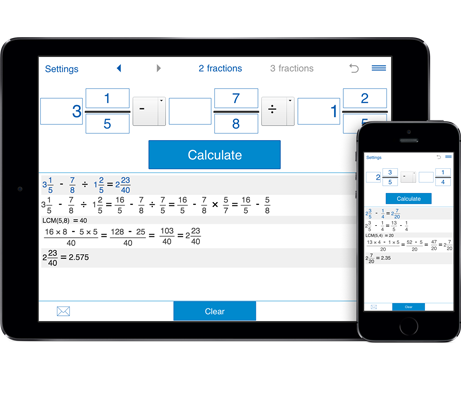# Fraction calculator with steps

This fraction calculator is ideal for solving various fraction problems and checking results. It shows you how to add, subtract, divide and multiply fractions, improper fractions and mixed numbers step-by-step.

## Calculate fractions

You can use fraction calculator for adding, subtracting, multiplying, and dividing two and three fractions. It not just calculates the results, but helps you understand how you got your answer. This way you can learn what you can't figure out yourself.
Fraction calculator is very simple to use. It lets you switch between "two fractions" and "three fractions" modes, easily input negative fractions and mixed numbers. Using the "Calculation history" option, you can easily to refer to your previous calculations.

Download the Intemodino Fraction calculator app for your computer or mobile device. Intemodino Fraction calculator is available for iOS, Android, Windows and Mac.

## How to use the fraction calculator

1. Select if you need to compute 2 or 3 fractions.

2. Enter the fractions in the provided fields. For proper and improper fractions, fill in the numerator and denominator fields. For mixed fractions, also fill the field corresponding to the whole part.

For negative fractions put a minus sign in front of a fraction.

3. Select the operation you wish to perform from the drop-down menu. If you calculate three fractions, you can combine operations.

4. Click "Calculate".

Related calculators:
To perform operations on more than three fractions, use our Fraction Calculator XL. Whether you are learning fractions or just need to check the results this is the app for you. It gives you step by step instructions on how to solve the problem.

To understand how to add, subtract, multiply and divide fractions, mixed numbers and decimals, try our Advanced fraction calculator